TowardsMachineLearning# What is Back Propagation through time (BPTT) in Recurrent Neural Network

## Introduction:-

In the post series of articles on Recurrent Neural Networks , In our last article , we’ve talked about what is RNN ( Recurrent Neural Network) ,Architecture of  RNN, Unfolding a Recurrent Neural Network, How RNN works ,Applications of RNNs in real life & Advantages of RNN . If you’ve not checked that out then I’ll request you to click here to read that article first .

• Back propagation in a Recurrent Neural Network or Back Propagation through time (  BPTT  )
• Long term dependencies  problem

## Back propagation in a Recurrent Neural Network or Back Propagation through time (BPTT ) :-

Back propagation is just a fancy name for Gradient descent .  It has some interesting properties but method behind it is exactly the same, just simply calculating the gradient and moving in that direction .

Similarly BPTT ( Back Propagation through time )  usually abbreviated as BPTT is just a fancy name for back propagation, which itself is a fancy name for Gradient descent . This is called BPTT because we are figuratively going back in time to change the weights, hence we call it the Back propagation through time(BPTT).

Recall from feed-forward Neural network , weights get updated via an error signal from whatever nodes it influences when it goes in forward direction.  Here I’ve shown the influence of  in purple, the upward arrows going in to the output Y only matter , if you’re considering that as a part of output . These will be different depending on whether you’ve a target for every time step or a target just at the end of the sequence . The arrows going from left to right must always be back- propagated because these are the hidden to hidden weights

So from above diagram , we’ve the following equations-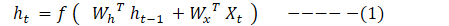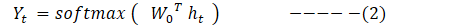### Note:- We’ve dropped the bias as of now to make pattern more obvious .

So using equation 1 & 2 we can rewrite the our neural network output as follows-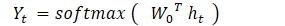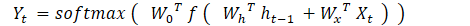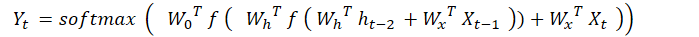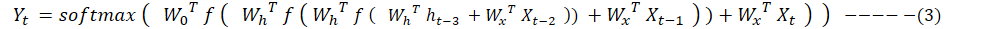let’s say we needed to go back in time 3 steps for Wh. So we need to use product rule from calculus  to find the output term.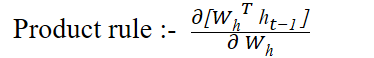One important thing that you want to try to see is that the same things are going to be multiplied over & over again due to chain rule of calculus . This will happen for both hidden to hidden weights as well as input to hidden weights.

The result is that you either get something that goes down to zero or something that gets very large quickly . These problems are called the vanishing gradients & exploding gradients problems respectively.

Let’s understand each of these separately.

From above equation (3 ) ,we can rewrite our output term in more generalized form as follows-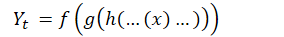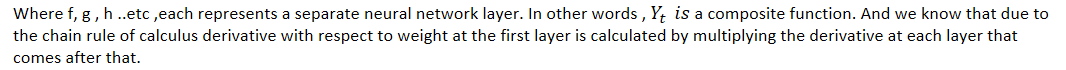So derivative at first layer can be written as follows-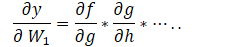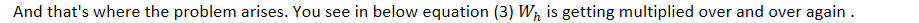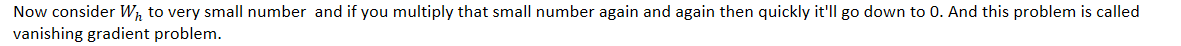If you look at the derivative of sigmoid then you must notice two things

• Derivative of sigmoid approaches 0 very quickly so by using the sigmoid a deep network or RNN is going to have a lot of derivatives very close to 0 causing it to learn very slowly
• Maximum value of sigmoid derivative is 0.25 . So even if we manage to get the peak value of the derivative at every layer , we’re still diminishing the magnitude of derivative by 25% at every layer .  So very deep neural networks just can’t be trained using standard back propagation.

Now of course we’ve deep neural networks and they just work fine. Another option is to use ReLu in lieu of Sigmoid . By using the ReLu , we can train the deep network using standard back propagation without any pretraining . Sometimes people call it end to end training.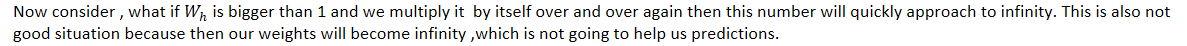So we now need our weights to be just right , neither too small nor too big. So for that to happen we need to initialize them to just right values. That’s where Weight initialization concept comes in play ,which we’ll discuss in another article.

It is a technique used to cope with the exploding gradient problem sometimes encountered when performing backpropagation. By capping the maximum value for the gradient, this phenomenon is controlled in practice.

## Long term dependencies problem:-

Sometimes we need access to recent information to predict next value. For example , consider a language model trying to predict the next word based on the previous ones. If we are trying to predict the last word in “Honesty is best policy,” we don’t need any further context – it’s pretty obvious the next word is going to be policy.

In such cases, where the gap between the relevant information and the place that it’s needed is small, RNNs can learn to use the past information.

But in real word scenarios , we’ve very long sequences and RNN doesn’t really perform good there. Let’s understand the problem with couple of examples-

### Example 1 :-  Consider the below text from Wikipedia about Albert Einstein.

By the time RNN reaches to end of paragraph , it might have forgotten about Albert Einstein’s “Theory of relativity” information.

### Example 2 :-

Now consider a scenario where we need more context  to predict the next value. Let’s consider a language model trying to predict last word in text like “I grew up in Spain… I speak fluent Spanish.” Recent information suggests that the next word is probably the name of a language, but if we want to narrow down which language, we need the context of Spain, from further back. It’s entirely possible that the gap between the relevant information and the point where it is needed to become very large.

Sometimes, we only need to look at recent information to perform the present task. For example, consider a language model trying to predict the next word based on the previous ones. If we are trying to predict the last word in “the clouds are in the sky,” we don’t need any further context – it’s pretty obvious the next word is going to be sky. In such cases, where the gap between the relevant information and the place that it’s needed is small, RNNs can learn to use the past information.

Unfortunately, as that gap grows, RNNs become unable to learn to connect the information.

The reason why it is difficult to capture long term dependencies is because of multiplicative gradient that can be exponentially decreasing/increasing ( Vanishing Gradient /Exploding Gradient) with respect to the number of layers.

Unfortunately, as that gap grows, RNNs become unable to learn to connect the information.

The reason why it is difficult to capture long term dependencies is because of multiplicative gradient that can be exponentially decreasing/increasing ( Vanishing Gradient /Exploding Gradient) with respect to the number of layers.

In theory, RNNs are absolutely capable of handling such “long-term dependencies.” A human could carefully pick parameters for them to solve toy problems of this form. Sadly, in practice, RNNs don’t seem to be able to learn them.

That’s where LSTM & GRU come to rescue.

## Downsides of RNNs:-

. These many recurrent units make the computation quite slow

• Long term dependencies (i.e. Difficulty of accessing information from a long time ago )
• Cannot consider any future input for the current state

So in next article we’ll discuss about LSTM & GRU.

## Article Credit:-

Name :  Praveen Kumar Anwla
Founder : TowardsMachineLearning .Org